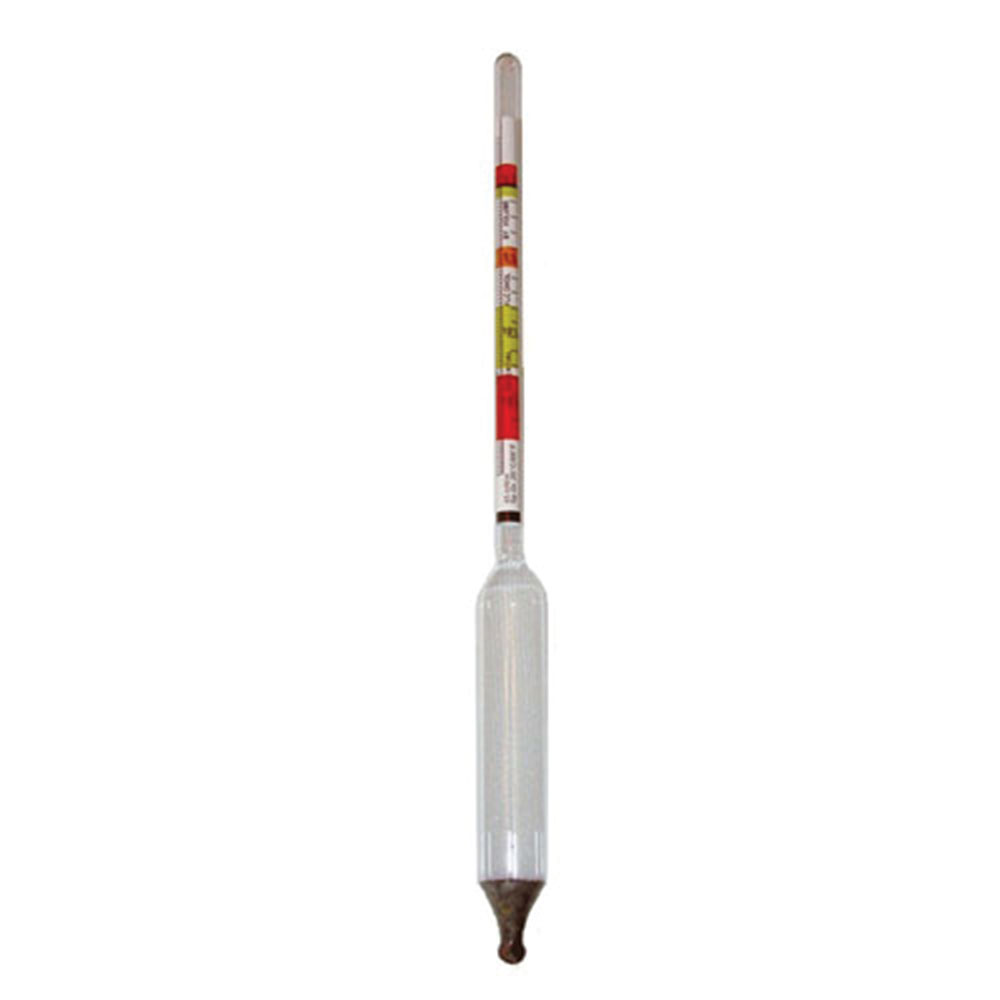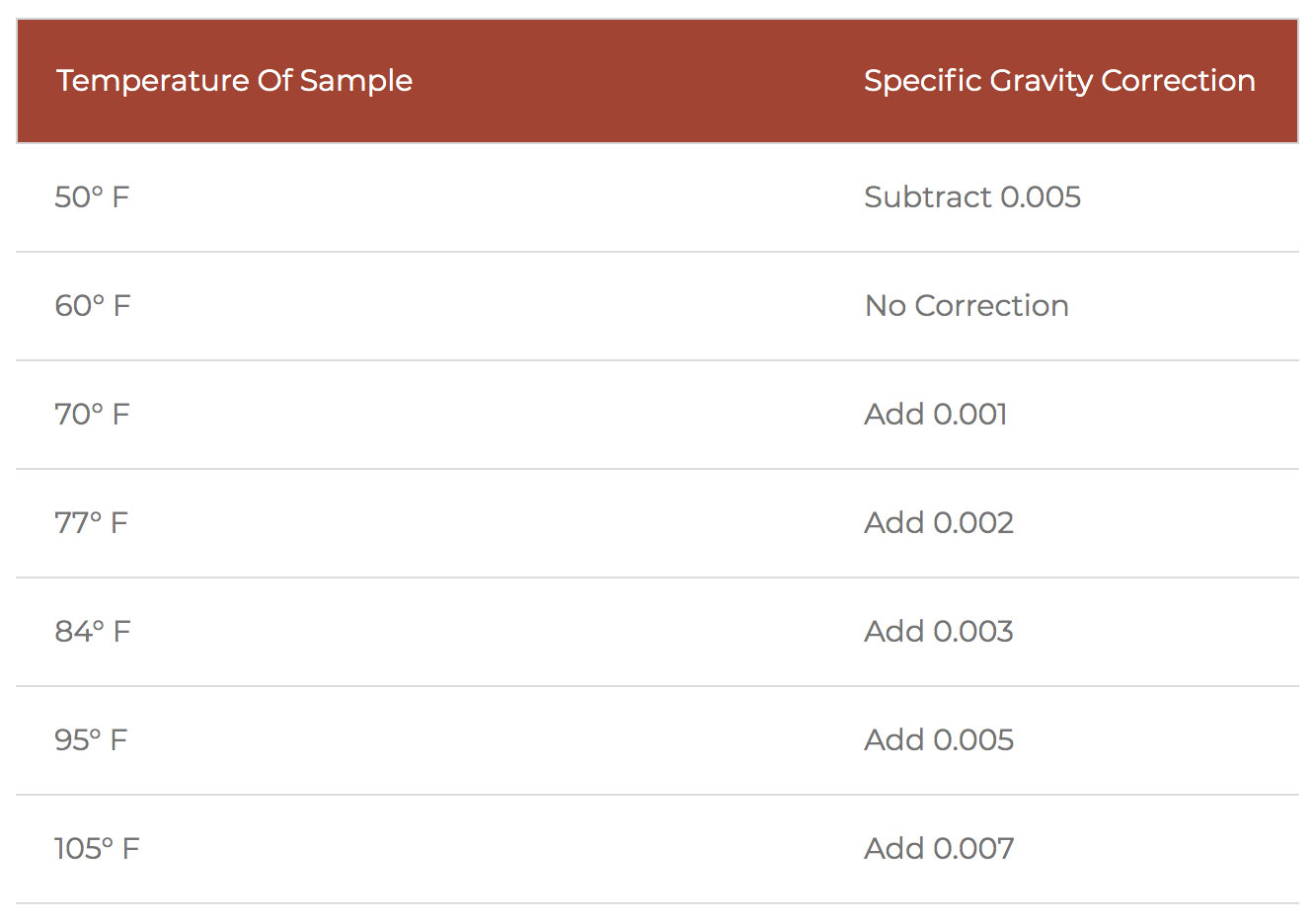# How To Use a Hydrometer

## Review Of The Hydrometer

First, let’s review the hydrometer. The hydrometer is a glass bulb that is weighted and floats in a testing jar. The hydrometer will tell you approximately how much alcohol was made in the fermentation process. The scale on the Mile Hi Distilling hydrometers is called a triple scale which gives you three scales. A brix reading, a specific gravity reading, and an approximate potential alcohol reading.

A hydrometer measures the density of a liquid in relation to water. In the case of distilling, we are measuring the amount of dissolved sugars in the wash or mash compared to water. Pure water has a specific gravity of 1.000 and as sugars are dissolved into solution it will cause the gravity to increase. So why do we need to use a hydrometer? A hydrometer gives you an estimated alcohol percentage that was produced in the fermentation process so you have an idea or estimate for how much alcohol there is to distill out.

If your fermentation did not work well and there was minimal alcohol produced in the fermentation then knowing what happened will help correct the problem. For example; maybe the yeast was pitched at too high of a temperature.

## The Three Scales On The Hydrometer

Brix is another way to measure sugar content and correlates with specific gravity. This hydrometer has a brix scale as well. A refractometer can also be used to  measure brix. A Brix value, is the number of grams of sucrose present per 100 grams of liquid. The value is measured on a scale of 1 to 100 and is used to calculate an approximate potential alcohol content by multiplying by 0.59. So, if a pre-fermented liquid measures 18 °Bx, its potential alcohol content will be approximately 10.6 percent ABV. This is estimated or an approximate value because there are other factors in the brewing and distilling process that can effect the efficiency.

### The Approximate Potential Alcohol Reading

This a good quick look at how much alcohol you will potentially produce if everything goes right in the fermentation process. FYI – the most alcohol you can create in fermentation is around 20%. What happens is when the alcohol gets around 20% the yeast cells die off and stop producing more alcohol.

Using this specific gravity reading when using a hydrometer is an estimated way to know how much alcohol was produced in fermentation. In order to accomplish this, you get a reading right at the start before you add yeast and you get another reading at the end of fermentation.

Use a simple math formulation and then you know how much estimated alcohol was created during fermentation so ultimately you have an idea of how much estimated alcohol there will be to distill out.. This gives you an estimate. Not every drop will be distilled out and also you will be removing foreshots, heads, and tails when you make your cuts.

See below step by step how to use a hydrometer using the specific gravity reading.

## Steps To Use a Hydrometer

### Step 1.

After the sugar water, mash, or wash has cooled to 70F to 80F and before you add the yeast fill up about 3/4 of your test jar (the plastic or glass test jar to float the hydrometer).  Float your hydrometer and write down the reading you get. This is the initial specific gravity or original specific gravity or called OG original gravity. The hydrometer is calibrated at 60F so there will need to be a temperature correction to get a more accurate reading. See temperature correction chart.

### Step 2.

Ferment for 5-7 days (fermentation times depends on what your fermenting) then check the specific gravity again which is now called the Final specific gravity or FG final gravity.### Step 3.

Now we need to do a little math so grab your calculator and complete the formula below:
OG (original gravity so the reading that you started with) subtract FG (final gravity so the reading that you ended with)
Take this number and multiply it by 131.25 to get your estimated percentage of alcohol in your fermentation.

### For Example:

OG (original specific gravity) of 1.080 subtract FG (final specific gravity) of 1.003 = .077 x 131.25 = 10.106% alcohol by volume (ABV)

So this example is telling us that there is estimated 10% alcohol made in this fermentation.

*Note:  Hydrometers can be used when fermenting grains, fruit, molasses, agave, etc.  There are temperature correction charts online if you want to get extremely accurate. For example if you check your wash at 70F compared to checking your wash at 75F there is  about ½ percent correction. A hydrometer is calibrated at 60F so if the temperature of the sample is different then see temperature correction chart.

Temperature Of Sample Specific Gravity Correction
50° F Subtract 0.005
60° F No Correction